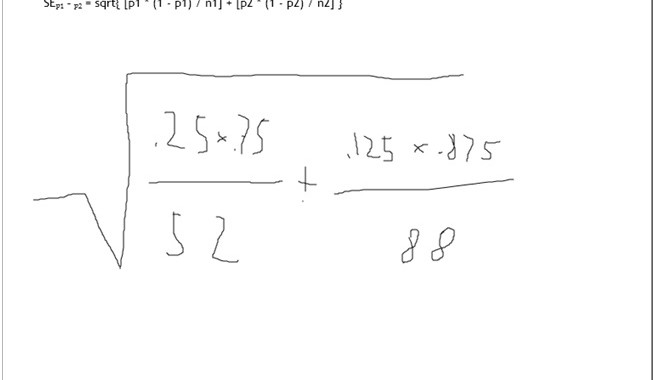Saltear al contenido principal# Error estándar en diferencia de porcentajes

Anahid nos muestra una excelente sesión de estadística, donde trataron el tema del error estándar.

Problema:Tutor

Welcome!  How are you?

Student

hello! good!

Tutor

Tell me, what can I do for you today?

Student

twell, im not sure how to find the standard error here for a difference in proportions

Tutor
ok, let’s find the proportions first, can you calculate them?

Student

.25 and .125

Tutor
Excellent!  You did that step perfectly. the formula for the SE is…
is that familiar to you?

Student
yeah, thats what i thought i originally did, i may have made a calculator error

Tutor
let’s check it together then. well done!
how much is the first term inside the square root?

Student

.0036

Tutor
perfect!

Student
and .0012

Tutor
well done, if we add them we get 0.00485. right?

Student
and then we square root for .069!

Tutor
perfect!
check that the square root is 0.0696

Student
yup! i got .0693 but i think its close enough

Tutor
if we take the first three digits after the dot, the approximation is 0.070
Great job on that one! Nicely done.

Student
actually, unrounded i did get 696, awesome, thank you for your help!

Tutor
you are welcome , Do you have any questions about anything we went over today?

Student
nope, thanks again!

Volver arriba